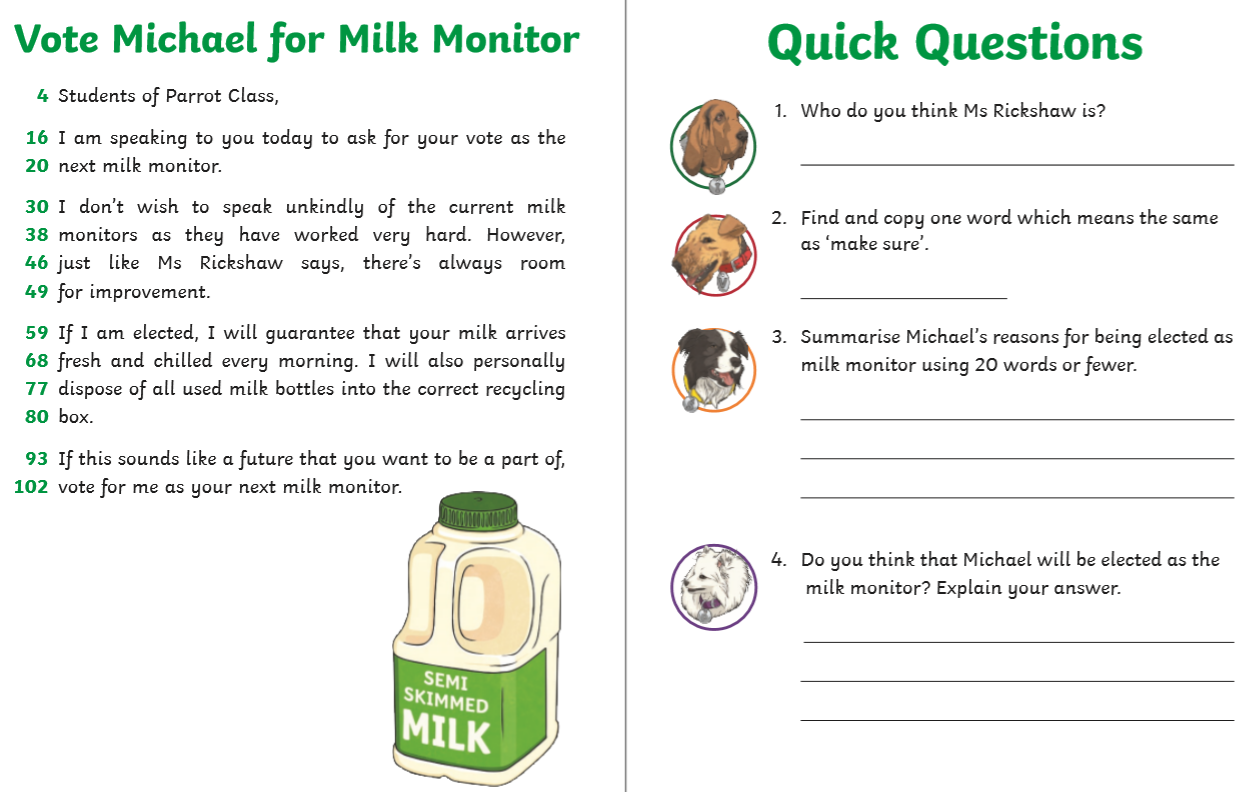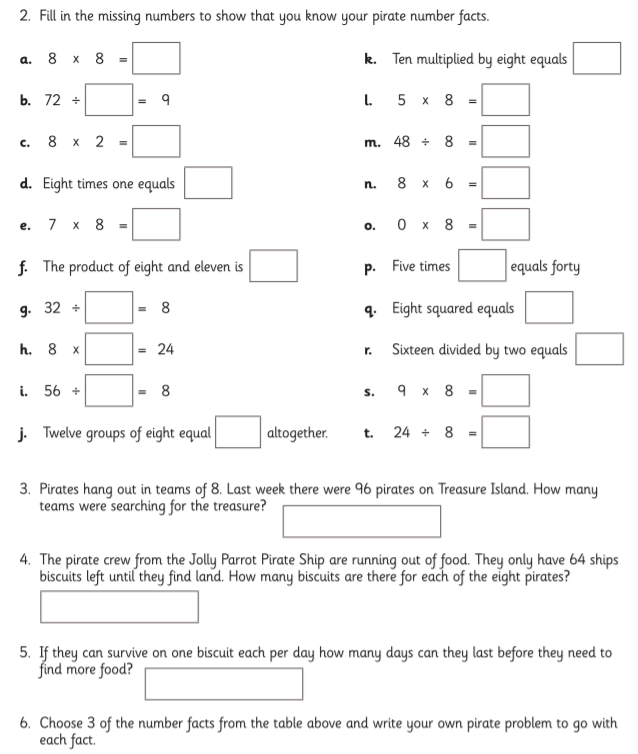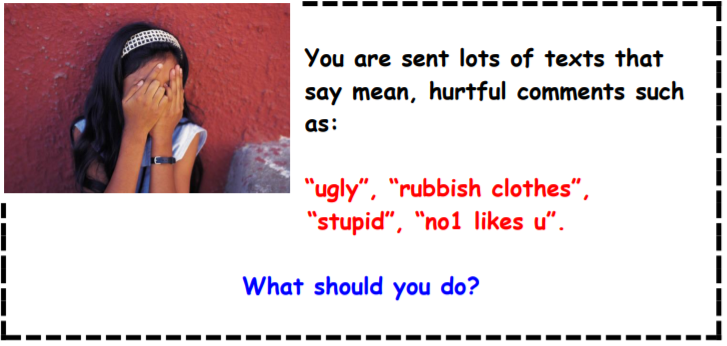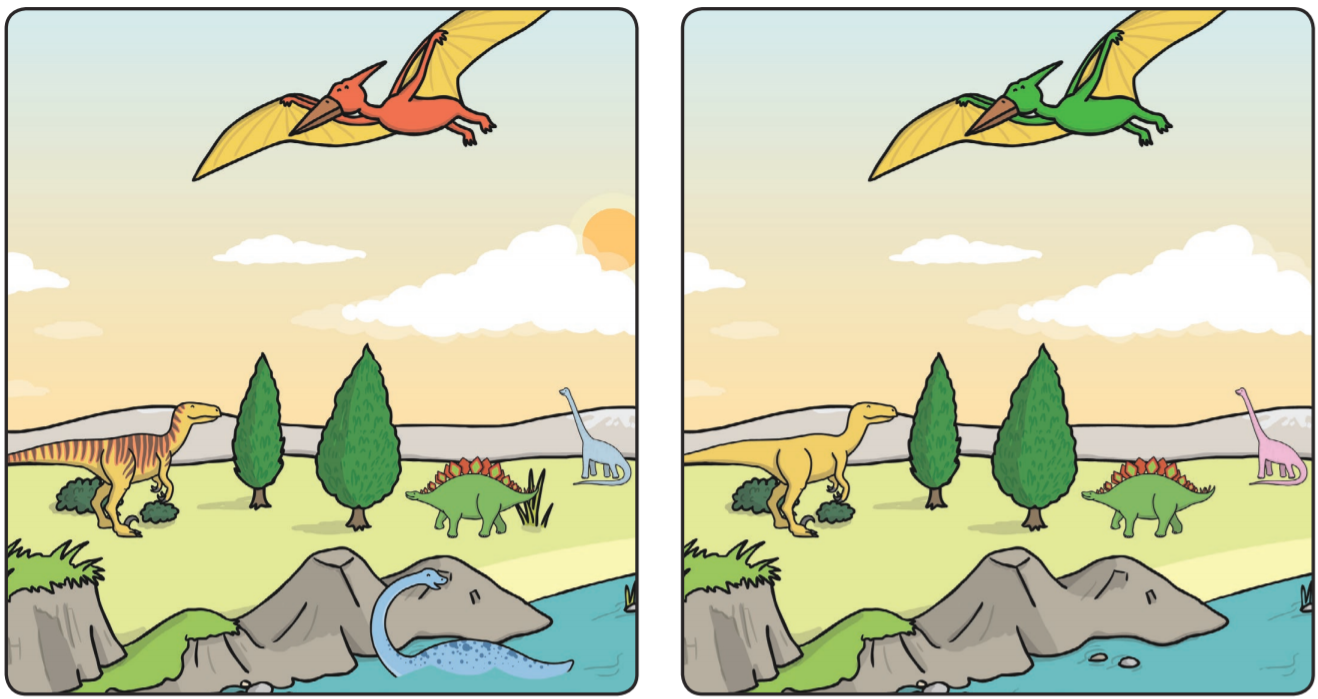# Year 3 – Friday 5th June 2020

Good morning everyone!

Well done on completing another week of home learning you’re all doing a fantastic job.

Thank you again for joining in with and completing the daily tasks on the blog.

If you are having a go at the tasks on paper or in books then do let us know what you’ve completed by commenting at the bottom of the page or email us some pictures of your work on yearthree@lingsprimary.org.uk

You might be in with a chance of a certificate next week!

Have a lovely weekend.## Maths:

Today’s video:

Today’s worksheets:

4s – multiples-of-4

8s –## Computing:# Finished? Have a go at these tasks too…

## Daily Riddle:

As it is Friday, the answers for today will be revealed on Monday so you need to check then to see if you have it correct. Involve everybody you can and see who is correct!

Here is today’s riddle: What has 13 hearts but no other organs?

## Spot the Difference:

Have a go at this spot the difference. There are 6 differences today. As it’s Friday the answers will be revealed on Monday so you need to check then to see if you have it correct.Today’s problem:## Hit the button:

We play this game in class all of the time, see how many of your times tables facts you can get right in 1 minute. Remember, in Year 3 you should know your 2, 3, 4, 5, 8 and 10 times tables.

https://www.topmarks.co.uk/maths-games/hit-the-button

## Story time:

No problem! Listening to a story is just as good as reading one.

Today, we are going to finish Charlie and The Chocolate Factory. There are 2 videos but only 3 chapters to listen to. We will be starting a new book next week. Let us know if you’ve enjoyed reading Charlie and The Chocolate Factory. Maybe you could write a review over the weekend?

### 7 thoughts on “Year 3 – Friday 5th June 2020”

•5th June 2020 at 10:30 am

1) I think Ms Rickshaw is a Milk Monitor.
2) Guarantee.
3) If i’m elected i guarantee that the milk arrives fresh.I’ll dispose all used milk bottles into correct recycling boxes.
4) Not sure.

Computing:
Tell an adult and forget it and delete it.

Daily Riddle:

What has 13 hearts but no other organs?

A deck of cards.

•5th June 2020 at 11:10 am

1) Ms Rickshaw is the class teacher

2) Make sure – guarantee

3) Micheal promises that milk arrives fresh and chilled every morning and used bottles are disposed of correctly.

4) I think Micheal will be elected because everyone likes fresh and chilled milk.

Maths:
2)
a) 8 x 8 = 64
b) 72 / 8 = 9
c) 8 x 2 = 16
d) eight times one equals eight
e) 7 x 8 = 56
f) The product of eight and eleven is 88
g) 32 + -24 = 8
h) 8 x 3 = 24
i) 56/9 = 8
j) Twelve groups of eight equal 96
k) Ten multiplied by eight equals 80
l) 5 x 8 = 40
m) 48 + 8 = 56
n) 8 x 6 = 48
o) 0 x 8 = 0
p) Five times 8 equals forty
q) Eight squared equals 64
r) Sixteen divided by two equals 8
s) 9 x 8 = 56
t) 24 / 8 = 3

3) 12 teams

4) 8 biscuits

5) 8 days

6)

Computing:

I will report to a trusted adult and not let it get to me.

Riddle:

A deck of cards

•5th June 2020 at 11:18 am

Miss rickshaw is a milk monitor
guarantee
if I am elected I guarantee that the milk will arrive fresh
yes I think he is going to get elected because he is kind

computer
tell a grown up and delete

•5th June 2020 at 11:55 am

1. I think that Ms Rickshaw is a teacher because they call her Ms and the teacher talk about her.
2. Guarantee
3. Michael will Guarantee the milk will arive fresh and chilled.
4. I think that Michael will be elected as the milk monitor because he is good at it.

•5th June 2020 at 12:06 pm

Q1) I think Ms. Rickshaw is the teacher of Parrot Class.
Q2) ” Guarantee” has the same meaning as ” make sure”.
Q3) Michael said that if he will be elected as a milk monitor, he will make sure that the milk arrives fresh and chilled every morning and also that he will personally dispose of all the used milk bottles.
Q4) Yes, I think Michael will be chosen a milk monitor because he will take care of the current milk situation really well based on the actions he promised to do to everyone voting.

COMPUTING:

MATHS:
2) Fill in the missing numbers to show that you know your pirate number facts.

a) 8 x 8 = 64
b) 72 divided by 8 = 9
c) 8 x 2 = 16
d) Eight times one equals eight.
e) 7 x 8 = 56
f) The product of eight and eleven is eighty eight.
g) 32 divided 4 = 8
h) 8 x 3 = 24
i) 56 divided by 7 = 8
j) Twelve groups of eight equals ninety six altogether.
k) Ten multiplied by eight equals eighty.
l) 5 x 8 = 40
m) 48 divided by 8 = 6
n) 8 x 6 = 48
o) 0 x 8 = 0
p) Five times eight equals forty.
q) Eight squared equals sixty four.
r) Sixteen divided by two equals eight.
s) 9 x 8 = 72
t) 24 divided by 8 = 3

3) There were 12 teams of pirates searching for treasure. 96 divided by 8 = 12
4) There are 8 biscuits lefts for each of the 8 pirates. 64 divided by 8 = 8
5) If 8 pirates were to have 1 biscuit each then they would survive for 8 more days. 8 divided by 1 = 8

•5th June 2020 at 1:32 pm

Maths
a.8 times 8 =64
b. 72 divied 8=9
c. 8 times 2= 16
d. 8 times1 =8
e.7 times 8 = 56
f. 8 times =88
g. 32 divided 5= 6
h. 8 times 4= 24
I. 56 divied 7= 8
j. 12 times 8 =96
k. 10 times 8 =80
l. 5 times 8 =40
m. 48 divided 8 =6
n. 8 times 6=48
o. 0 times 8= 0
p. 5 times 8= 40
q. 8 times 8=64
r. 16 divided 2 =8
s. 9 times 8 =72
t. 24 divided 8 =4

1. I think she is th monitor
2. Guarantee is the word as make sure
4. Yes because he made a promise

daily riddle:
Deck of cards.

•